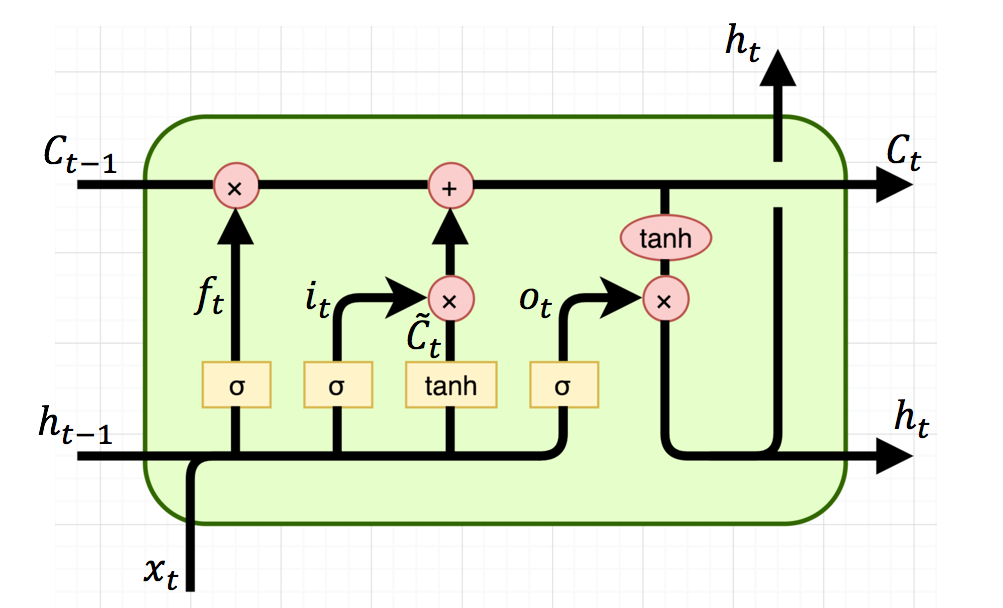# Simple RNN vs GRU vs LSTM :- Difference lies in More Flexible control

When we start reading about RNN (Recurrent Neural Net) and its advanced cells, we are introduced with a Memory Unit (in GRU) and then additional Gates (in LSTM).

However, if we analyse the basic architecture of all 3,Basic architectures of RNN, GRU and LSTM cells

we can easily see, there is no such Memory Unit present in the cells. But increasing number of Mathematical operations of Input(xt) and previous output (ct-1, ht-1).

Simple RNN :- Here there is simple multiplication of Input (xt) and Previous Output (ht-1). Passed through Tanh activation function. No Gates present.

Gated Recurrent Unit (GRU) :- Here a Update gate is introduced, to decide whether to pass Previous O/P (ht-1)to next Cell (as ht) or not. Forget gate is nothing but additional Mathematical Operations with a new set of Weights (Wt).

But, Math operations are performed on same inputs (i.e. xt and ht-1).

Long Short Term Memory Unit (LSTM) :- Here 2 more Gates are introduced (Forget and Output) in addition to Update gate of GRU. And again as above, these are additional Mathematical Operations on same inputs (xt and ht-1). So overall, LSTM has introduced 2 Math operations having 2 new sets of Weights.

# Conclusion :-

Considering above explanation, if we represent these Mathematical operations by Taps/Control Nobs.

We can say that, when we move from RNN to LSTM, we are introducing more & more controlling knobs, which control the flow and mixing of Inputs as per trained Weights. And thus, bringing in more flexibility in controlling the outputs.

So, LSTM gives us the most Control-ability and thus, Better Results. But also comes with more Complexity and Operating Cost.

Data Engineer with Telecom Experience

## More from Saurabh Rathor

Data Engineer with Telecom Experience